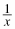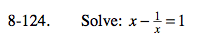### Home > PC > Chapter 8 > Lesson 8.2.4 > Problem8-124

8-124.

Solve: x= 1 Homework Help ✎1. Multiply each term by x.
2. You now have a quadratic. Put all terms on one side and set = 0.
3. Factor or use the Quadratic Formula to solve.

$x=\frac{1\pm \sqrt5}{2}$